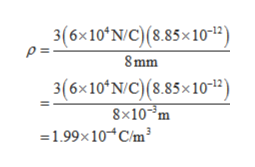The electric field is measured for points at distances r from the center of a uniformly charged insulating sphere that has volume charge density ρ and radius R, where r<RPart A Calculate ρ.

Question

The electric field is measured for points at distances r from the center of a uniformly charged insulating sphere that has volume charge density ρ and radius R, where r<R

Part A
Calculate ρ.

Step 1

Electric field is given by,

Step 2

Re-arranging and substituting the v...help_outlineImage Transcriptionclose3(6x10 N/C)(8.85x10-12) P= 8mm 3(6x10 N/C) (8.85x1012 8x10-3m 1.99x10 C/m3 fullscreen

Want to see the full answer?

See Solution

Want to see this answer and more?

Our solutions are written by experts, many with advanced degrees, and available 24/7

See Solution
Tagged in

Electric Charges and Fields